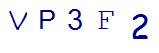# Back to School Basics: What Parents Need to Know About 5th Grade MathLast Updated on August 31, 2021 by Thinkster

Fifth grade: For some kids, it is the start of middle school. For others, it is the last year of elementary. No matter how your district divides students, a close look at Common Core State Standards and typical math concepts covered in various schools show that 5th grade math is a considered challenging. Here’s what you need to know about what your child is facing this year.

### Writing and Interpreting Expressions

In 5th grade math, kids start preparing for a deeper understanding of algebraic concepts. Common Core requires them to be able to write and understand numerical expressions using parentheses, brackets or braces. They should also be able to use equations to represent word problems and write simple equations with variables. The basic properties of addition and multiplication are also required.

### Analyzing Patterns and Relationships

Students in 5th grade math are going to spend a lot of time analyzing and working with tables and graphs. They will begin graphing linear functions and using coordinate graphs, and will work with function tables as well. They also need to be able to find patterns, continue them, and graph the results.

### Number Operations in Base Ten

By fifth grade, students should be well-versed in recognizing the place-value system and identifying values in specific places. They also should be able to multiply, divide, subtract and add. In fifth grade, they will begin working even more with decimals and will be making the connection between decimals and the fraction equivalents. Furthermore, they will also learn scientific notation and will work with decimals and fractions in expanded form. Lastly, rounding and estimating with decimals is also important.

This is not the first year students will be multiplying and dividing, but in 5th grade math, the numbers are going to get larger. You will also find that the word problems associated with multiplication and division become increasingly complex, with multiple steps often required.

### Working with Fractions

This is the year when work with fractions becomes intense. Students will be learning how to do various operations with fractions and mixed numbers, including adding and subtracting with unlike denominators. Borrowing and carrying with fractions is also taught in fifth grade. Students will also be asked to use real-world examples and models to help with fractions.

5th grade geometry will include coordinate graphs and graphing points on a plane. Students will need to be able to classify quadrilaterals and work with both regular and irregular polygons.

Geometry and measurement will be working closely together in 5th grade math, as students will be finding volume and area of shapes and prisms of many different sizes. They will also be comparing and converting units of measurement from one unit to the next.

Does this list seem overwhelming to you? Are you wondering whether or not you remember all of these concepts? If you want to give your child the best chance of success at 5th grade math, even with limited knowledge on your part, then consider an online tutoring option that will give them the boost they need.

Thinkster Math offers tablet-based tutoring that your child can tap into whenever you have some spare time. Created by teachers with today’s math standards in mind, Thinkster provides instruction at the point of learning for greater success. This can be the tool that you need to give your child a chance at success with challenging 5th grade math concepts.

SummaryArticle Name
Back to School Basics: What Parents Need to Know About 5th Grade Math
Description
If your child is about to start 5th grade, there are some things you need to be prepared for. Here's what you can expect with 5th grade math.
Author
Publisher Name
Thinkster Math
Publisher Logo

## Subscribe to Thinkster Blog## Recommended Articles### Unlocking the Code: Teaching Mathematical Symbols and Their Meaning

Knowledge of mathematical symbols is a foundational skill that empowers students to engage with and excel i...